# Map scale + area - math problems

#### Number of problems found: 15

• A rectangle 8A rectangle measuring 6 cm and 4 cm is enlarged by the ratio 3:1. What is the area of the enlarged rectangle?
• Hectares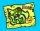Determine the area of the rectangular land (in ha), which has dimensions of 3 cm and 4.5 cm on the plan with a scale of 1: 40,000.
• A mapA map with a scale of 1: 5,000 shows a rectangular field with an area of 18 ha. The length of the field is three times its width. The area of the field on the map is 72 cm square. What is the actual length and width of the field?
• Scale of planOn the plan of the village in the scale of 1: 1000 a rectangular garden is drawn. Its dimensions on the plan are 25mm and 28mm. Determine the area of the garden in ares.
• Map scaleThe rectangular plot has a scale of 1: 10000 area 3 cm2 on the map. What content does this plot have on a 1:5000 scale map?
• Map scaleOn a 1:1000 scale map is a rectangular land of 4.2 cm and 5.8 cm. What is the area of this land in square meters?
• The land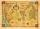The land in the shape of a square has 9 ha. How big a page will the land have at the scale of 1: 5000?
• PlotThe plot on which Mr. Kalous is to build a house has the shape of a rectangle. On a 1: 500 scale, its dimensions are 7cm and 5.5cm. Find out the dimensions of the plot. Calculate the parcel size.
• Map scaleGarden has on plan on a scale of 1: 150 width 22 cm and length 35 cm. What is the real area of the garden?
• Square garden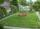On the plan with a scale of 1:1500 is drawn as a square garden with area 81 cm2. How many meters is long garden fence? Determine the actual acreage gardens.
• Plan of the villagePlan of the municipality in 1:1000 scale has plotted garden with dimensions 25 mm and 28 mm. Determine the area of gardens in ares in reality.
• Hřiště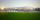Map scale is 1: 5000. The playground is rectangular and on the map has dimensions 10 cm and 5 cm What is area of playground in square meters in reality?
• ScaleThe swimming pool is long 110 m and 30 m wide. The plan of the city is shown as a rectangle with an area 8.25 cm2. What scale is the city plan?
• FloorThe floor area of ​​the room is 31 m2 and has a width of 4.3 m. How many centimeters of circumference measured the floor on the map at the scale 1:75?
• Map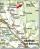Forest has an area of ​​36 ha. How much area is occupied by forest on the map at scale 1:500?

Do you have an exciting math question or word problem that you can't solve? Ask a question or post a math problem, and we can try to solve it.

We will send a solution to your e-mail address. Solved examples are also published here. Please enter the e-mail correctly and check whether you don't have a full mailbox.

Map scale - math problems. Area - math problems.